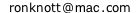# 2016

2016 - some of the more unusual, fascinating and fun facts about the number.
Some are left as puzzles for you to solve with a to show the solutions.
The facts below are all derived from Neil Sloane's On-line Encyclopedia of Integer Sequences where the sequences containing 2016 listed here are shown as, for instance,
A224950
which is also a link to the series itself.

First some fun facts about the year 2016 and then about the number 2016.

## The year 2016

2016 is a leap year with 53 Fridays and 53 Saturdays.
A224950

There are 5 Mondays in February this year. The last time that happened was 1988 and the next is 2044.
A135795

The transit of Mercury across the sun will be visible from somewhere on the earth this year.
The next time is in 2019.
A171466.

## The number 2016

### What 2016 looks like

Small: Large: Very Large: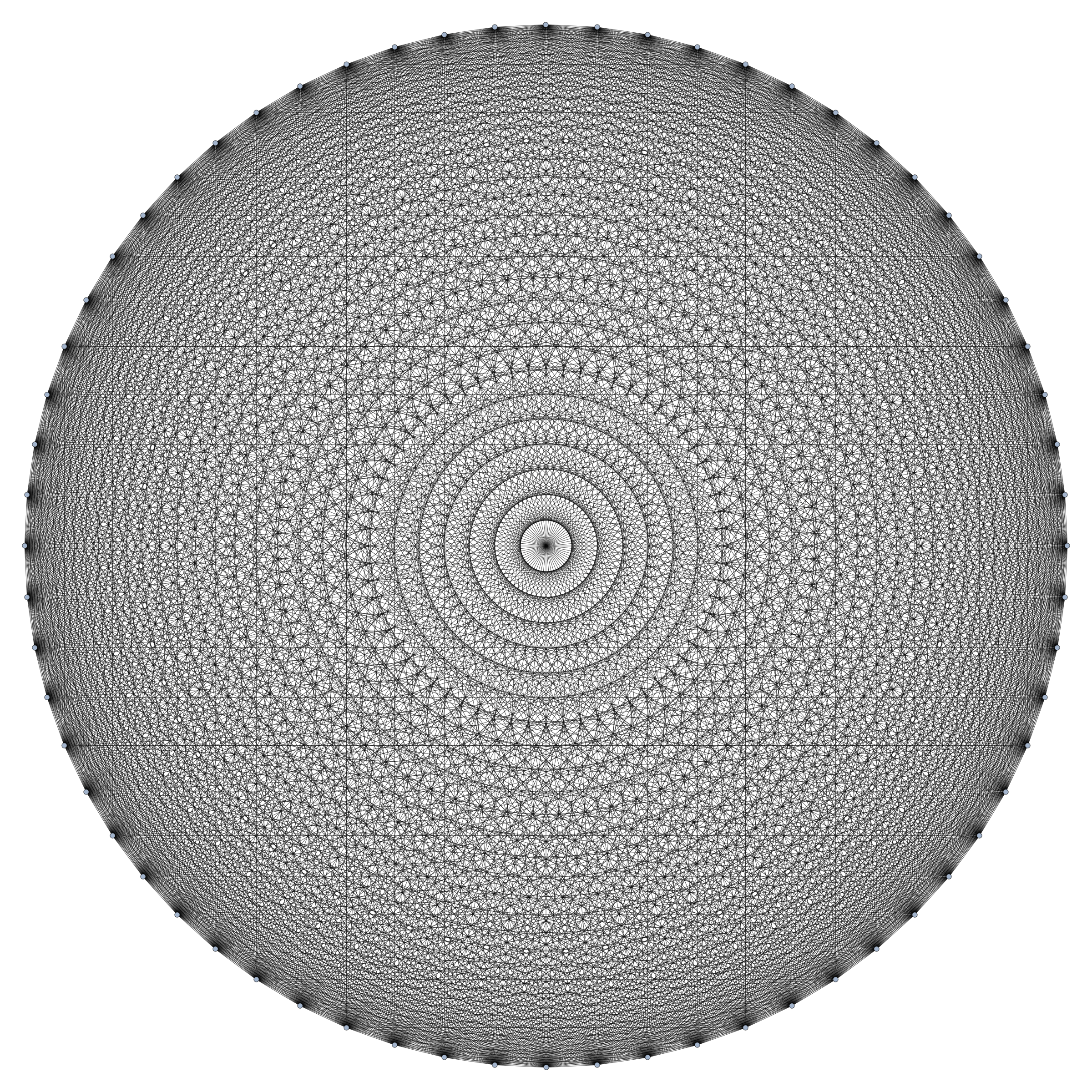If we draw 64 points round a circle then join each to every other, there will be 64×63/2 = 2016 lines.
Here it is and it must surely be the prettiest way to see 2016!

### Fun ways to write 2016

Alex Bellos in his Monday Puzzle of 4 January 2016 in the Guardian newspaper posed the problem of using the numbers 1 to 10 and various maths operations to make 2016. Surely the most elegant (to quote Alex and I agree) is the following from James Annan and others:
 10×9×8×7×6 = 2016 5+4+3+2+1
It turns out that this is just one of a whole pattern where we add the first n numbers on the bottom and multiply the next n on the top.
 2 = 2, 4×3 = 4, 6×5×4 = 20, ... 2n×(2n-1)×...×(n+2)×(n+1) 1 2+1 3+2+1 n+(n-1)+...+2+1

It is an easy exercise to show that they will all be whole numbers.
A110371

### In different Bases

In binary (base 2) 2016 is 11111100000 with six 1s followed by five 0s.
The next number with this pattern of a block of 1s followed by a block of 0s is 2032 which is 11111110000 in binary.
A006516, A043569

Also, the digit sum of the binary number 11111100000 is 6 which is a factor of 2016.
If we write 2016 in every base from 2 to 10 and sum the digits each time, we will always get a total that is itself a divisor of 2016.

Base2016 in base Digitsum2016/Digitsum
2111111000006336
322022008252
41332009224
5310318252
6132006336
7561012168
8374014144
9268016126
1020169224
We can go beyond base 10 if we represent the digits in bases beyond 10 as their decimal values
for example, 2016 = 11×132 + 12×13 + 1 = 11 12 1 then this property of 2016 extends for several more bases yet. How many?
Base2016 in base Digitsum2016/Digitsum
111 5 7 3 16 126
121 2 0 0 3 672
1311 12 12484
1410 4 014144
158 14 62872
167 14 02196
176 16 103263
For base 18, 201618=6 4 0 with digitsum 10 but 2016 is not a multiple of 10.

### With Squares ...

There are 12 ways to write 2016 as a difference of two squares. What are they?
452 − 32 = 2016
462 − 102 = 2016
502 − 222 = 2016
542 − 302 = 2016
652 − 472 = 2016
712 − 552 = 2016
792 − 652 = 2016
902 − 782 = 2016
1302 − 1222 = 2016
1712 − 1652 = 2016
2542 − 2502 = 2016
5052 − 5032 = 2016

Now find three whole numbers a, b and c, all different, such that a2 − b2 = b2 − c2 = 2016.
a=79, b=65, c=47
472 = 2209, 652 = 4225 and 792= 6241 have 2016 as the difference between one and the next.

A256418

How many ways can you write 32 as a sum of 7 squares if 0 and repetitions are allowed and also each different order of the 7 squares counts as a different answer? There are a total of 2016 solutions!
That is too many to list by hand so try this simpler problem :
find the 6 ways of writing 32 as a sum of 7 squares, 0 and repetitions being allowed, if the numbers to be squared are written in order of size. For example,
02 + 02 + 02 + 02 + 02 + 42 + 42 = 32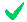but we do not count 02 + 02 + 02 + 02 + 42 + 02 + 42 = 32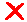because 0,0,0,0,4,0,4 is not in the correct order.

Each of these is a basic solution:
02 + 02 + 02 + 02 + 02 + 42 + 42 = 32
02 + 02 + 12 + 12 + 12 + 22 + 52 = 32
02 + 02 + 12 + 22 + 32 + 32 + 32 = 32
02 + 02 + 22 + 22 + 22 + 22 + 42 = 32
02 + 12 + 12 + 12 + 22 + 32 + 42 = 32
12 + 12 + 22 + 22 + 22 + 32 + 32 = 32
A045849

Can you find 4 whole numbers a, b, c and d, all in arithmetic progression (when written in order each differs from the next by the same amount) and the sum of their squares is 2016?

a=16, b=20, c=24, d=28
162 + 202 + 242 + 282 = 2016

### ... and Cubes

33 + 43 + 53 + 63 + 73 + 83 + 93 = 2016
A217843

### Runsums

A Runsum is a sum of a run of consecutive integers:
2016 = 1 + 2 + 3 + ... + 63
2016 = 86 + 87 + ... + 106
2016 = 220 + 221 + ... + 228
2016 = 285 + 286 + ... + 291
2016 = 671 + 672 + 673
Runsums

### Factors

2016 = 2×2×2×2×2×3×3×7 = 32×63 = 26−1(26−1)

2016 = 16 ×126: 2016 is the smallest multiple of 16 (after 16 itself) that ends 016
What is the next multiple of 16 ending 016?

4016 = 251 × 16

2016 = 8 × 252
If we reverse the digits in 8 and 252, we get the same numbers. But also
2016 = 24 × 84 = 42 × 48
where if both factors are again reversed the new numbers still have the same product 2016!
So 2016 has two separate pairs of factors that, when the individual factors are reversed, they still have a product of 2016.
What is the smallest number with two pairs of factors like this?
What is the next such number after 2016?

The smallest number with two such pairs of factors is 484 and the next after 2016 is 2178:
484 = 11×44 = 22×22
2178 = 22×99 = 33×66
If we exclude the self-reversing factor pairs such as 8 × 252 which give the same pair when they are reversed, then see A228164

If we take a list of the numbers from 1 to 4, we can find 16 subsets of them: {}, {1}, {2}, {3}, {4}, {1, 2}, {1, 3}, {1, 4}, {2, 3}, {2, 4}, {3, 4}, {1, 2, 3}, {1, 2, 4}, {1, 3, 4}, {2, 3, 4}, {1, 2, 3, 4}
Take each subset and multiply all the numbers in the subset. Some products will be repeated but there are only 8 different products.
If we try this with the numbers from 1 to 14, there are exactly 2016 different products.
A060957

215 = 32768 where the product of the digits is 3×2×7×6×8 = 2016.
A014257
What is the next year that is a product of the digits of a power of 2?

2700
216 = 65536 and 6×5×5×3×6 = 2700

## Pythagorean Triangles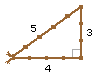A Pythagorean Triangle is a right-angled triangle whose sides are whole numbers, such as sides 3,4 and 5.
Once we have one such triangle, we can always multiply all the sides by 2 say to get 6-8-10 or by 3 to find 9-12-15 and so on. All these have the same shape.
If we want different shapes then the smallest Pythagorean triangle of each shape is called a primitive Pythagorean triangle or PPT for short. All Pythagorean triangles are PPTs or a multiple of a PPT.
There is a whole series of PPTs where the longest side, the hypotenuse, is just 1 more than one of the other sides as here in the 3-4-5 triangle. The next is 5-12-13, and the series continues 7-24-25, 9-40-41, 11-60-61, 13-84-85, 15-112-113. If we add up the areas of these 7 triangles we have a total area of 2016.

The Pythagorean triangle 32-126-130 which has sides double those of the PPT 16-63-65 has an area 2016.

A083374
A055112: the areas
Pythagorean Triangles

### Other Integer-sided triangles

You can find exactly 11 integer-sided triangles (not necessarily right-angled) which have an area of 2016.
Six of them are 52-80-84, 48-85-91, 58-70-96, 65-72-119, 32-126-130 (right-angled), 64-225-287.
In 64-225-287, the two sides 64=82 and 225=152 are themselves square numbers.
What are the other five integer-sided triangles with an area of 2016?
52-80-84 perimeter=216
48-85-91 perimeter=224
58-70-96 perimeter=224
65-72-119 perimeter=256
32-126-130 perimeter=288 Pythagorean
64-225-287 perimeter=576

60-112-164 perimeter=336
20-204-208 perimeter=432
35-192-221 perimeter=448
56-170-222 perimeter=448
109-148-255 perimeter=512

A051586
A070150
A232461

## Polygonal Numbers

In maths we have square numbers 12=1, 22=4, 32=9, ... , each a number multiplied by itself. But a square number is so called because that number of dots can be arranged in a square shape.
 side shape size 1 2 3 4 5 6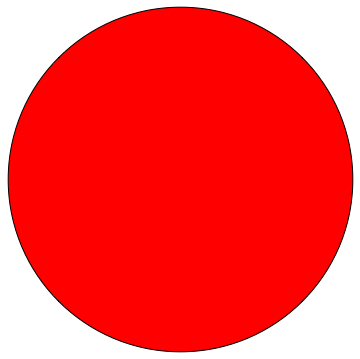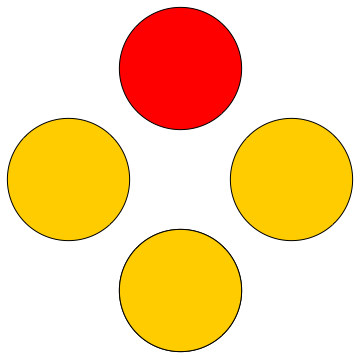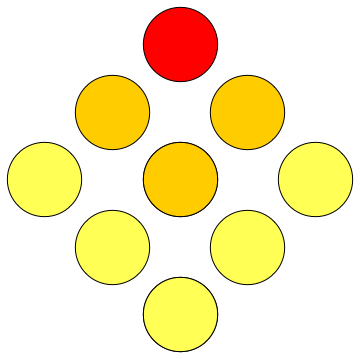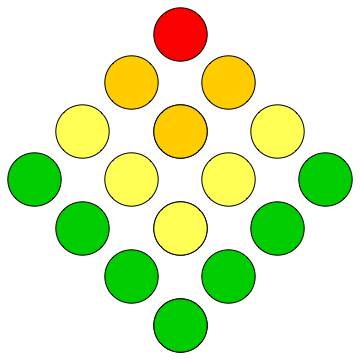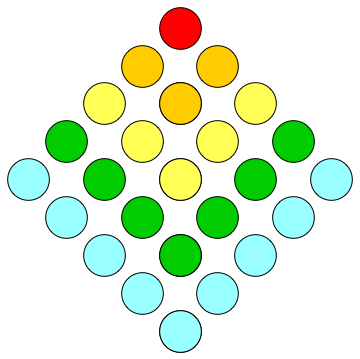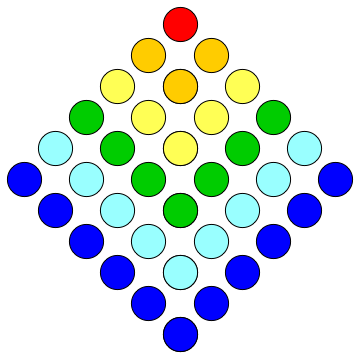1 4 9 16 25 36
Other shapes are triangular numbers 1, 1+2=3, 1+2+3=6,
 side shape size 1 2 3 4 5 6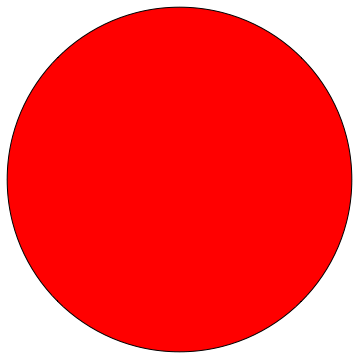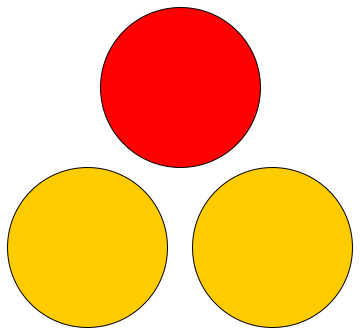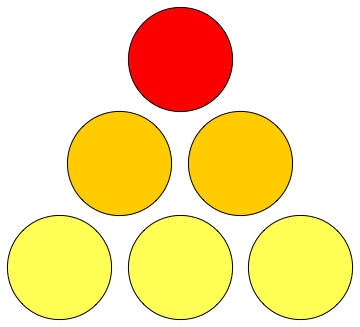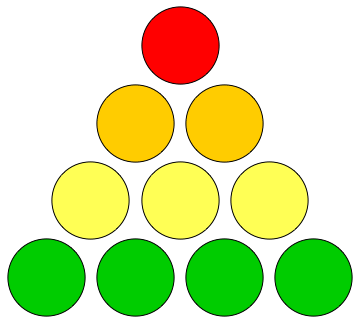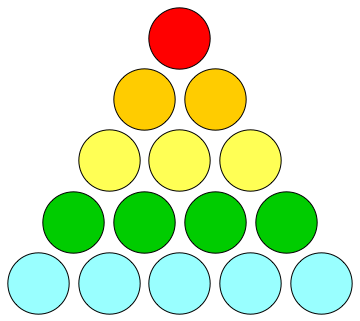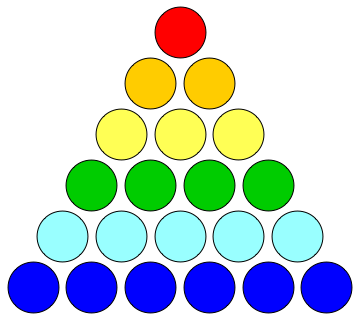1 3 6 10 15 21
... and pentagonal, hexagonal and so on.
• 2016 is 63rd triangular number: 1 + 2 + ... + 63 = 2016
• 2016 is the smallest Triangular number divisible by 32
A066561
• 2016 has 36 divisors and 36 is also a Triangular number. (The next number with this property is 3321 with 10 divisors.)
A116541
A076172
• 2016 is the 32nd hexagonal number: 1 + 6 + 15 + 28 + ... + 378 = 2016
• 2016 is the 14th 24-gonal number
• 2016 is the sixth 136-gonal number
• 2016 is the third 673-gonal number.
Triangular numbers: A000217,
Hexagonal numbers: A000384,
24-gonal numbers: A051876.

## Dominoes on a Pyramidal Board

If we take a board of squares arranged in a pyramid shape, we can place a domino on it either horizontally: or vertically: to cover two squares on the board.
The first board here has 4 squares on its base. There are just 6 ways to place a domino on the squares: 4 with it horizontal and 2 verticallly.
If the pyramid board has a base of 32 squares and is 16 levels tall, we can place a domino on it in 2016 ways.
A000384

## A Magic Square of Prime Numbers

 15 6 1 8 7 5 3 2 9 4
 79 137 197 199 277 347 349 431 127 193 131 419 337 421 107 281 103 379 283 389 293 227 179 163 397 251 83 271 269 157 439 149 409 211 383 191 181 101 401 139 307 239 317 167 89 367 97 433 353 233 359 151 257 223 331 109 241 373 263 229 313 173 113 311
A Magic square is a square of whole numbers with every row, column and both diagonals having the same sum, called the magic constant for the square.
On the left is a magic square using the numbers 1 to 9 and each row, column and diagonal sums to 15.

We can use all 64 consecutive prime numbers between 79 and 439 to make an 8x8 magic square with magic constant 2016. This is the smallest magic constant in an 8x8 square of consecutive primes.

A073520
A189188
Found by G Abe and A Suzuki, two "amateur" mathematicians and reported in The Study of Magic Squares G Abe, 1957 (Japanese only).
See prime puzzles and problems page.

## More on Squares

### Painting walls

In a 9x7 cm rectangle of squares, if we trace around every square of every size then we would have drawn a line 2016 cms long.
There are 63 of size 1x1, some of size 2x2, and so on, up to the three of size 7x7.
For each size from 1x1 to 7x7, calculate how many squares there are of that size and therefore how long the perimeter is of all the squares of each size and so verify that the total is 2016.

 There are 9×7 = 63 squares of size 1×1 each with perimeter 4×1 = 4 cm There are 8×6 = 48 squares of size 2×2 each with perimeter 4×2 = 8 cm There are 7×5 = 35 squares of size 3×3 each with perimeter 4×3 = 12 cm There are 6×4 = 24 squares of size 4×4 each with perimeter 4×4 = 16 cm There are 5×3 = 15 squares of size 5×5 each with perimeter 4×5 = 20 cm There are 4×2 = 12 squares of size 6×6 each with perimeter 4×6 = 24 cm There are 3×1 = 3 squares of size 7×7 each with perimeter 4×7 = 28 cm
That's a total of 63×4+48×8+35×12+24×16+15×20+12×24+3×28 = 2016 cm.

### Squares in a circle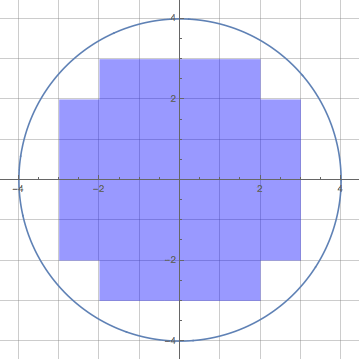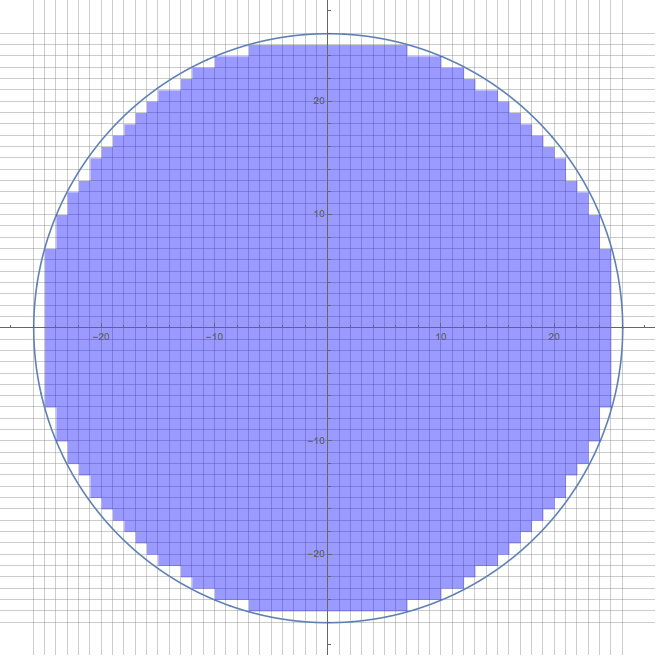Click on the image
to enlarge or reduce
If we draw a circle of radius 4 on graph paper, we find there are 32 complete squares inside the circle.
There are 2016 squares completely inside a circle of radius of 26.
A119677 are the alternate terms of A136485. Also see A261849.

## Hypercubes

The 9-dimensional hypercube of 512 vertices and 2304 edges has 2016 5-dimensional hypercubes (penteracts).
A135273
Hypercubes are the continuation of the series: a point, a line, a square, a cube into 4 and more dimensions.
Each is derived from the previous one by taking a copy of it but imagining that all the points leave a trail from the original to the copy, forming new edges in the new dimension.
 Shape Dimension ∙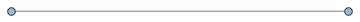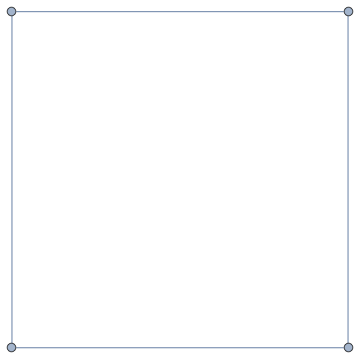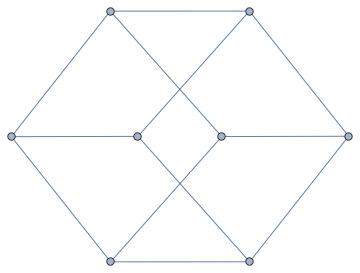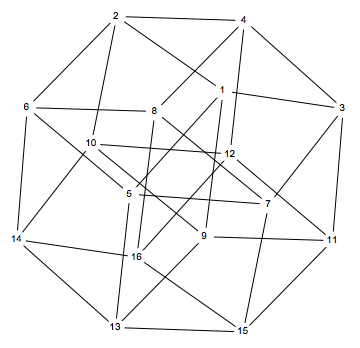0 1 2 3 4
The number of points will double each time we go to the next dimension.
The number of edges will double but also there are extra edges between the pairs points between the original and the copy.
The square faces and cubes are more difficult to see but the formula is as follows:
we have taken two copies of the previous dimensional object so we double the number of faces (cubes, ...) etc. But we also have made new edges, faces, etc equal to the number of 1-dimension-smaller objects, so the extra faces are made from each edge in the smaller dimension, the extra cubes are made form each face in the smaller dimension and so on.
So from a cube with 8 points and 6 square faces and 12 edges to the next dimension, we find the faces are 2×6 faces and 12 square faces from the 12 edges: a total of 24 square faces in the 4D hypercube.
Similarly, the number of edges in the 4D hypercube cube is twice 12 edges from the two cubes plus 8 more where the 8 points of the cube now become edge in the 4D version: a total of 24+8=32 edges.
ShapeDimensionpointsedgesfacescubes4D5D6D7D8D9D
Point 01
Line 121
Square 2 441
Cube 3 81261
4D Hypercube4 16 32 24 8 1
5D Hypercube5 32 80 80 40 10 1
6D Hypercube6 64 192 240 160 60 12 1
7D Hypercube7 128 448 672 560 280 84 14 1
8D Hypercube8 256 1024 1792 1792 1120 448 112 16 1
9D Hypercube9 512 2304 4608 5376 4032 2016 672 144 18 1

© 1 January 2016 (updated 5 February 2016)Dr Ron Knott